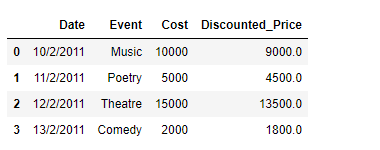Related Articles
Create a new column in Pandas DataFrame based on the existing columns
• Last Updated : 23 Jan, 2019

While working with data in Pandas, we perform a vast array of operations on the data to get the data in the desired form. One of these operations could be that we want to create new columns in the DataFrame based on the result of some operations on the existing columns in the DataFrame. Let’s discuss several ways in which we can do that.

Given a Dataframe containing data about an event, we would like to create a new column called ‘Discounted_Price’, which is calculated after applying a discount of 10% on the Ticket price.

Solution #1: We can use `DataFrame.apply()` function to achieve this task.

 `# importing pandas as pd ` `import` `pandas as pd ` ` `  `# Creating the DataFrame ` `df ``=` `pd.DataFrame({``'Date'``:[``'10/2/2011'``, ``'11/2/2011'``, ``'12/2/2011'``, ``'13/2/2011'``], ` `                    ``'Event'``:[``'Music'``, ``'Poetry'``, ``'Theatre'``, ``'Comedy'``], ` `                    ``'Cost'``:[``10000``, ``5000``, ``15000``, ``2000``]}) ` ` `  `# Print the dataframe ` `print``(df) `

Output :Now we will create a new column called ‘Discounted_Price’ after applying a 10% discount on the existing ‘Cost’ column.

 `# using apply function to create a new column ` `df[``'Discounted_Price'``] ``=` `df.``apply``(``lambda` `row: row.Cost ``-`  `                                  ``(row.Cost ``*` `0.1``), axis ``=` `1``) ` ` `  `# Print the DataFrame after addition ` `# of new column ` `print``(df) `

Output :Solution #2: We can achieve the same result by directly performing the required operation on the desired column element-wise.

 `# importing pandas as pd ` `import` `pandas as pd ` ` `  `# Creating the DataFrame ` `df ``=` `pd.DataFrame({``'Date'``:[``'10/2/2011'``, ``'11/2/2011'``, ``'12/2/2011'``, ``'13/2/2011'``], ` `                    ``'Event'``:[``'Music'``, ``'Poetry'``, ``'Theatre'``, ``'Comedy'``], ` `                    ``'Cost'``:[``10000``, ``5000``, ``15000``, ``2000``]}) ` ` `  `# Create a new column 'Discounted_Price' after applying ` `# 10% discount on the existing 'Cost' column. ` ` `  `# create a new column ` `df[``'Discounted_Price'``] ``=` `df[``'Cost'``] ``-` `(``0.1` `*` `df[``'Cost'``]) ` ` `  `# Print the DataFrame after  ` `# addition of new column ` `print``(df) `

Output :Attention geek! Strengthen your foundations with the Python Programming Foundation Course and learn the basics.

To begin with, your interview preparations Enhance your Data Structures concepts with the Python DS Course.

My Personal Notes arrow_drop_up
Recommended Articles
Page :